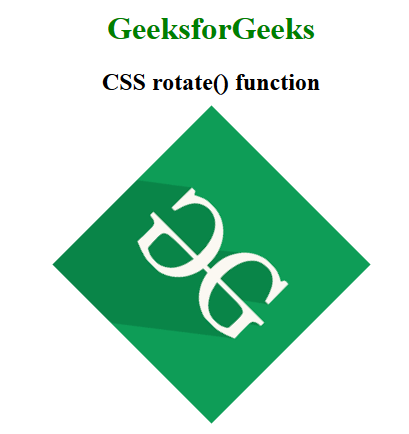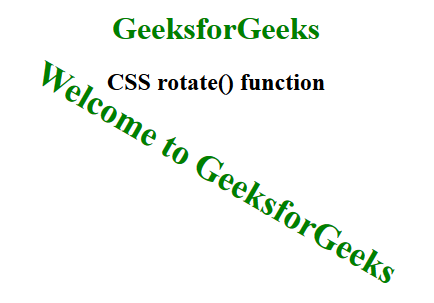# CSS rotate() Function

The rotate() function is an inbuilt function which is used to rotate an element based on the given angle as an argument. The angle can be set in terms of degrees, gradians, radians, or turns.

Syntax:

`rotate( angle )`

Parameters: This function accepts single parameter angle which represents the angle of rotations. The positive and negative angles rotate the elements in clockwise and counter-clockwise respectively.

Below examples illustrates the rotate() function in CSS:

Example 1:

## html

 `  ` `<``html``>  ` `<``head``>  ` `    ``<``title``>CSS rotate() function  ` `    ``<``style``>  ` `        ``body { ` `            ``text-align:center; ` `        ``} ` `        ``h1 { ` `            ``color:green; ` `        ``} ` `        ``.rotate_image { ` `            ``transform: rotate(45deg); ` `        ``} ` `    ``  ` `  ` ` `  `<``body``>  ` `    ``<``h1``>GeeksforGeeks ` `    ``<``h2``>CSS rotate() function ` `    ``<``br``><``br``> ` `     `  `    ``<``img` `class``=``"rotate_image"` `src``=  ` `"https://media.geeksforgeeks.org/wp-content/cdn-uploads/20190710102234/download3.png"` `        ``alt``=``"GeeksforGeeks logo"``>  ` `  ` ` `

Output:Example 2:

## html

 `  ` `<``html``>  ` `<``head``>  ` `    ``<``title``>CSS rotate() function  ` `    ``<``style``>  ` `        ``body { ` `            ``text-align:center; ` `        ``} ` `        ``h1 { ` `            ``color:green; ` `        ``} ` `        ``.GFG { ` `            ``font-size:35px; ` `            ``font-weight:bold; ` `            ``color:green; ` `            ``transform: rotate(30deg); ` `        ``} ` `    ``  ` `  ` ` `  `<``body``>  ` `    ``<``h1``>GeeksforGeeks ` `    ``<``h2``>CSS rotate() function ` `    ``<``br``><``br``> ` `     `  `    ``<``div` `class``=``"GFG"``>Welcome to GeeksforGeeks  ` `  ` `                      `

Output:Supported Browsers: The browsers supported by rotate() function are listed below: